# Posts Tagged ‘ UsingR ’

## Using R for Introductory Statistics 6, Simulations

March 21, 2011
By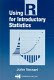R can easily generate random samples from a whole library of probability distributions. We might want to do this to gain insight into the distribution's shape and properties. A tricky aspect of statistics is that results like the central limit theore...

## Using R for Introductory Statistics, The Geometric distribution

March 13, 2011
By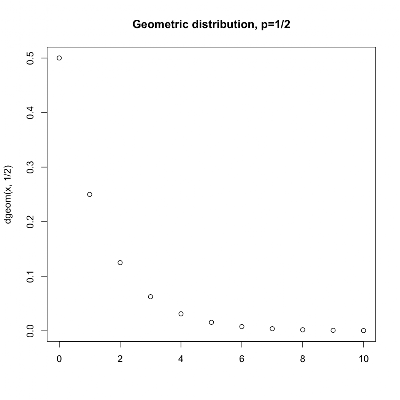We've already seen two discrete probability distributions, the binomial and the hypergeometric. The binomial distribution describes the number of successes in a series of independent trials with replacement. The hypergeometric distribution describes th...

## Using R for Introductory Statistics, Chapter 5, hypergeometric distribution

February 21, 2011
By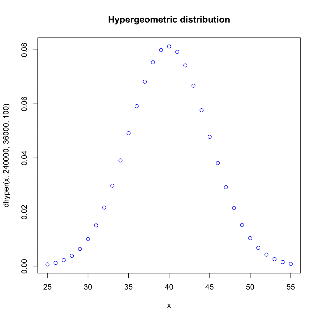This is a little digression from Chapter 5 of Using R for Introductory Statistics that led me to the hypergeometric distribution. Question 5.13 A sample of 100 people is drawn from a population of 600,000. If it is known that 40% of the population h...

## Using R for Introductory Statistics, Chapter 5, Probability Distributions

February 9, 2011
By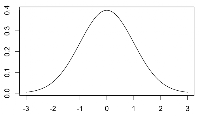In Chapter 5 of Using R for Introductory Statistics we get a brief introduction to probability and, as part of that, a few common probability distributions. Specifically, the normal, binomial, exponential and lognormal distributions make an appearance....

## Using R for Introductory Statistics, Chapter 5

January 23, 2011
ByAny good stats book has to cover a bit of basic probability. That's the purpose of Chapter 5 of Using R for Introductory Statistics, starting with a few definitions: Random variable A random number drawn from a population. A random variable is ...

## Using R for Introductory Statistics, Chapter 4, Model Formulae

January 10, 2011
By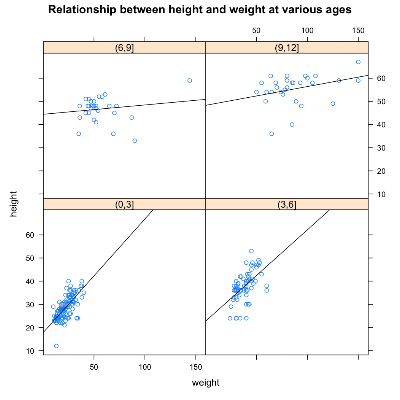Several R functions take model formulae as parameters. Model formulae are symbolic expressions. They define a relationship between variables rather than an arithmetic expression to be evaluated immediately. Model formulae are defined with the tilde ope...

## Using R for Introductory Statistics, Chapter 4

December 12, 2010
ByChapter 4 of Using R for Introductory Statistics gets us started working with multivariate data. The question is: what are the relationships among the variables? One way to go about answering it is by pairwise comparison of variables. Another techniq...

## Using R for Introductory Statistics, Chapter 3.4

August 21, 2010
By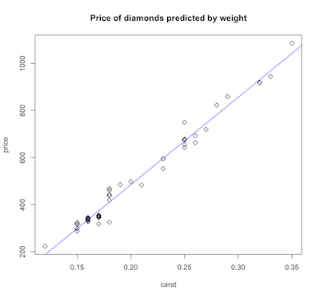...a continuing journey through Using R for Introductory Statistics, by John Verzani. Simple linear regression Linear regression is a kooky term for fitting a line to some data. This odd bit of terminology can be blamed on Sir Francis Galton, a proli...

## Using R for Introductory Statistics 3.3

August 11, 2010
By...continuing our way though John Verzani's Using R for introductory statistics. Previous installments: chapt1&2, chapt3.1, chapt3.2 Relationships in numeric data If two data series have a natural pairing (x1,y1),...,(xn,yn), then we can ask, &ld...

## Using R for Introductory Statistics 3.2

June 6, 2010
By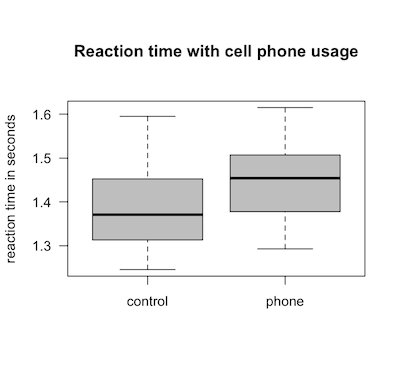...continuing my sloth-like progress through John Verzani's Using R for Introductory Statistics. Previous installments: Chapters 1 and 2 and 3.1. Comparing independent samples Boxplots provide a visual comparison between two or more distributions. Fo...